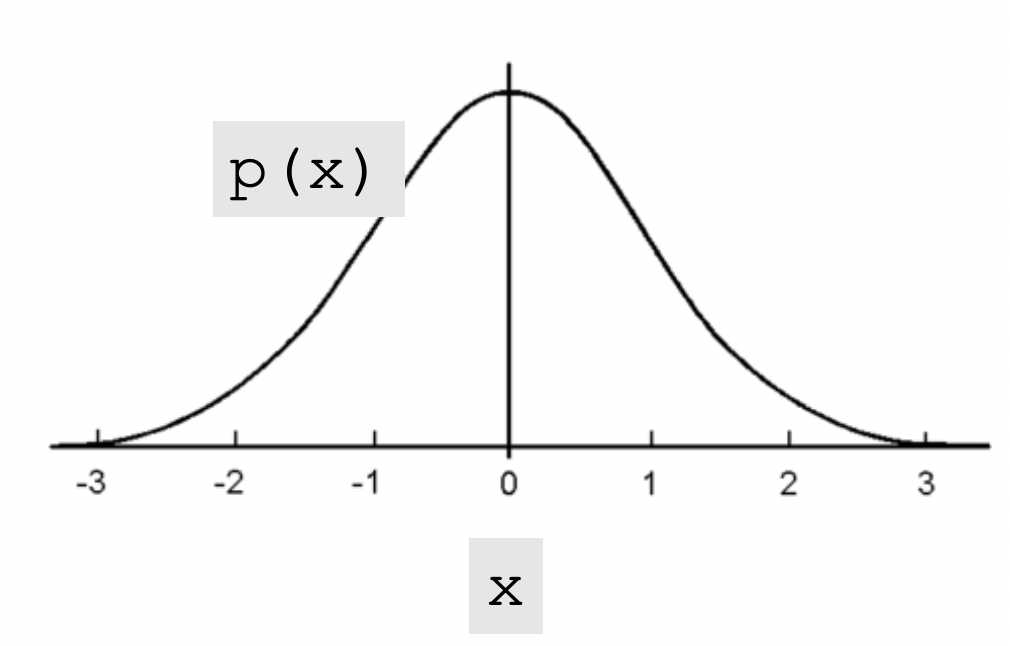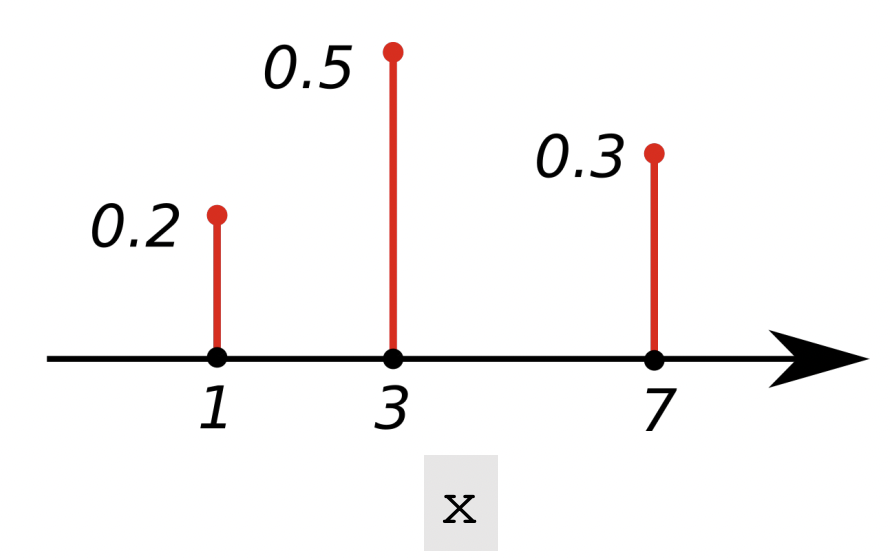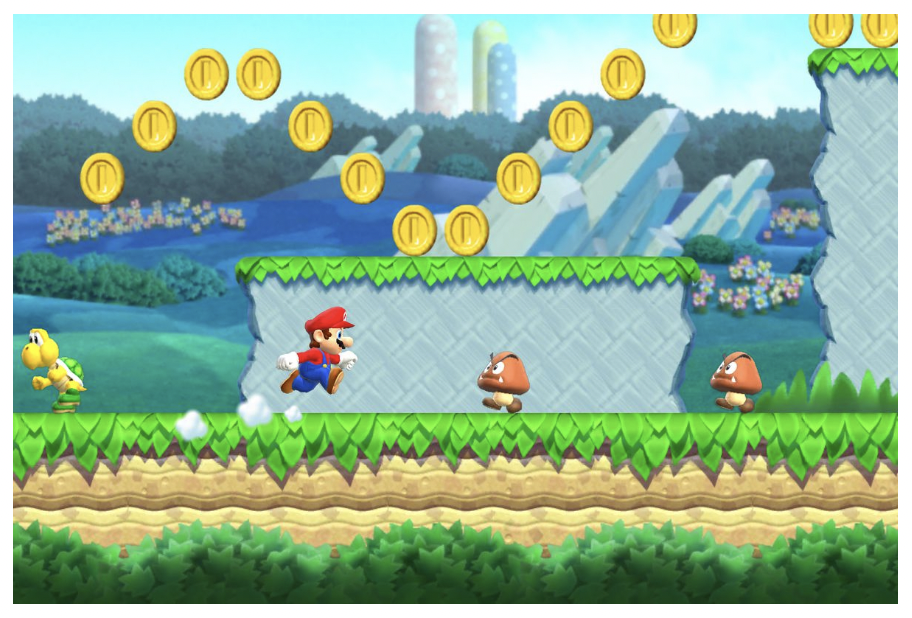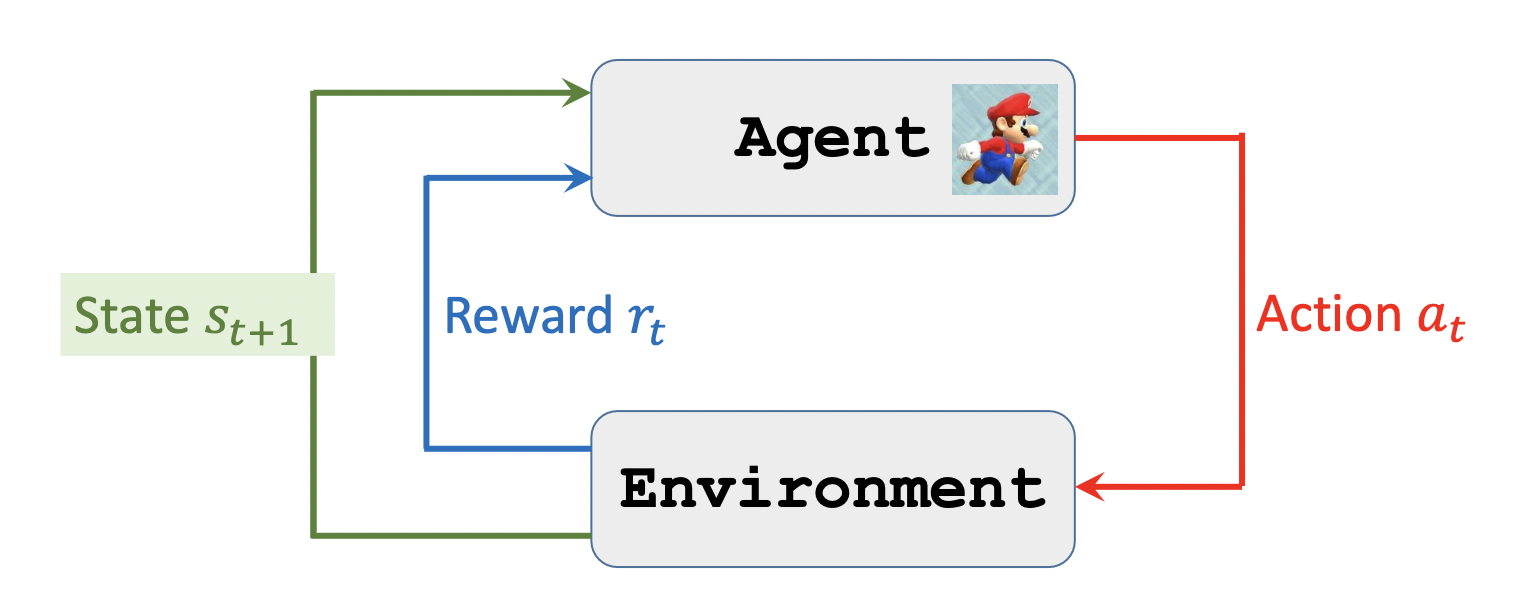Shusen Wang

## 基础

Probalility Density Function-PDFPDF：$p(1)=0.2, p(3)=0.5, p(7)=0.3$$\int_x p(x) d x=1$

$\sum_{x \in x} p(x)=1$

Expectation

## 术语$s$ state: 游戏中的一帧图片。

$a$action: 马里奥可以左，右，跳三个动作。 所以$a \in\{$ left, right, up $\}$

$\boldsymbol{\pi}$policy function:策略函数，根据当前的state选择最好的action。$\pi(a \mid s)=\mathbb{P}(A=a \mid S=s)$

$R$Reward:吃一个金币，或赢得游戏。赢得游戏的奖赏大于吃一个金币。触碰到Goomba则奖赏为负数，上述所有情况都没发生则奖赏为0.

state transition:## 随机性

$A \sim \pi(\cdot \mid s)$

old state 根据action转变到new state。$S^{\prime} \sim p(\cdot \mid s, a)$状态也是随机的。

## 定义action会产生一个新的state和Reward

Definition ： Return 累计未来奖励(cumulative future reward)。

$U_t=R_t+R_{t+1}+R_{t+2}+R_{t+3}+\cdots$

Definition：Discounted return

$\gamma$:discount factor(超参数)

$U_t=R_t+\gamma R_{t+1}+\gamma^2 R_{t+2}+\gamma^3 R_{t+3}+\cdots$随机的，R依赖于s，a。 s，a是随机的。

Definition： Action-Value Function 为动作A打分。当前动作好不好

$Q_\pi\left(s_t, a_t\right)=\mathbb{E}\left[U_t \mid S_t=s_t, A_t=a_t\right]$条件期望

$U_t$依赖于$S_t,S_{t+1},...,S_n$和$A_t,A_{t+1},...,A_n$

$s_t,a_t$是观测变量，$s_{t+1},...,S_n$和$A_{t+1},...,A_n$是随机变量

Actions are random：$\mathbb{P}[A=a \mid S=s]=\pi(a \mid s)$ policy function

State are random：$\mathbb{P}\left[S^{\prime}=s^{\prime} \mid S=s, A=a\right]=p\left(s^{\prime} \mid s, a\right)$ state function

Ut也是随机变量。

Definition： Optimal action-value function

$Q^{\star}\left(s_t, a_t\right)=\max _\pi Q_\pi\left(s_t, a_t\right)$去掉policy function。最优动作价值函数

Definition：State-value function

$V_\pi\left(s_t\right)=\mathbb{E}_A\left[Q_\pi\left(s_t, A\right)\right]=\sum_a \pi\left(a \mid s_t\right) \cdot Q_\pi\left(s_t, a\right)$当Aciton是离散的时候。

End